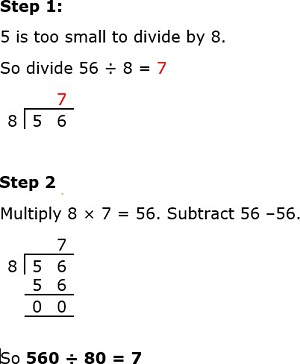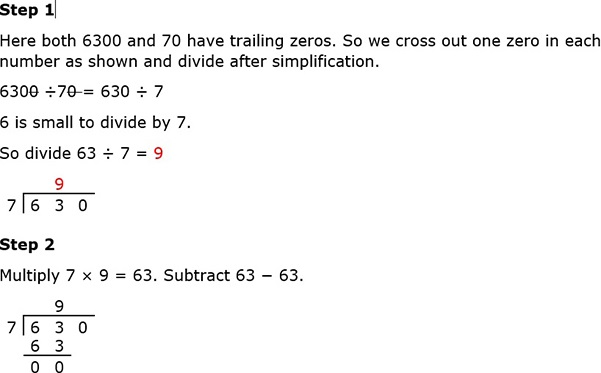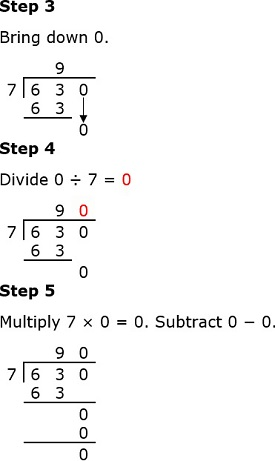# Division with trailing zeros: Problem type 2

In this type of problems, for example, divide 6400 ÷ 40, the dividend and the divisor both have trailing zeros. So, before starting the division, their quotient is simplified by removing the same number of zeros at the end of each number.

Divide 560 ÷ 80

### Solution

Here both 560 and 80 have trailing zeros. So before starting the division, we simplify by removing the same number of zeros at the end of each number as follows.

560 ÷ 80 = 56 ÷ 8

The simplified division is now 56 ÷8Divide 6300 ÷ 70

### SolutionSo, 6300 ÷ 70 = 90